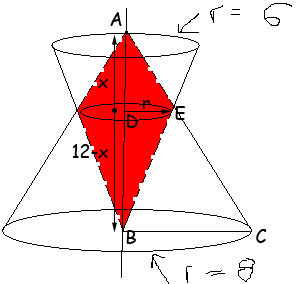SEARCH HOMEMath Central Quandaries & QueriesI am in Advanced Geometry class in 9th grade and cannot solve this problem that is due on January 2cd! If you could please solve this and provide work to support your answer i would greatly appreciate it. I have included an attachment with a diagram for the problem. Thanks in advance! A cone of radius 6 and height 12 and a different cone of radius 8 and height 12 intersect as shown in the figure below, where the vertex of one matches with the center of the base of the other. Find the volume of the intersection of the two cones (in exact form). Provide work to support your answer.Cassie,

I took the diagram you sent and added two dimensions, r for the radius of the common base of the two red cones and x for the height of the top red cone. The bottom red cone has height 12 - x.Triangles ABC and ADE are similar so

12/8x/r and hence 3r = 2x

A second pair of similar triangles will give you a second equation relating x to r.

PennyMath Central is supported by the University of Regina and The Pacific Institute for the Mathematical Sciences.